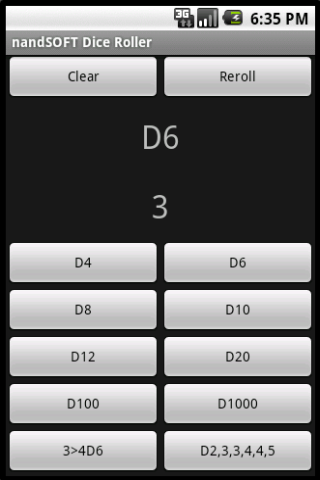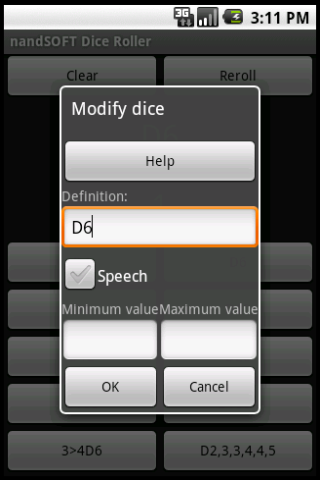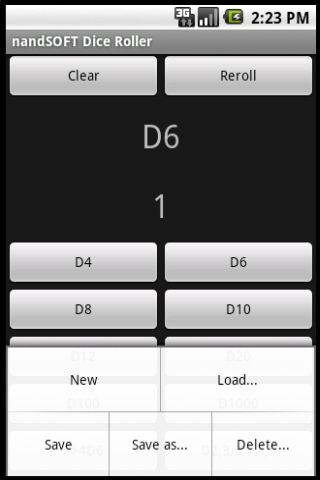Dice RollerDice syntax

You can use the four operators + - * / and parenthesis ( ) for basic operations.

xDy roll x dice with y faces. Example, the sum of two six-side dice:

2D6

xBy roll x times button y (1-10). Example, the sum of the two dice defined in the 4th button:

2B4

n>xDy take n higher dice from a pool of x dice with y faces. Example, the sum of the three higher six-side dice from a roll of four dice:

3>4D6

n<xDy take n lower dice from a pool of x dice with y faces. Example, the sum of the two lower ten-side dice from a roll of three dice:

2<2D10

xDa,b_c for single specific values (or ranges). Example, if you want to roll two dice with 2, 3, 3, 4, 4, 5 numbers:

2D2,3,3,4,4,5

Example, if you want to roll a die with number from 1 to 10 and 20:

D1_10,20

xDa,b,cP for adding a die to the last value (open roll). Example, if you obtain a 6 on a D6 add another die:

D1,2,3,4,5,6P

xDMa,b,c for subtracting a die to the first value. Example, if you obtain a 6 on a D6, add nother die, and if you obtain a 1, subract another die:

DM1,2,3,4,5,6P

You can roll multiple dice and showing them side by side using the semicolon ; separator. Example, a six-side die roll and a four-side die roll:

D6;D4